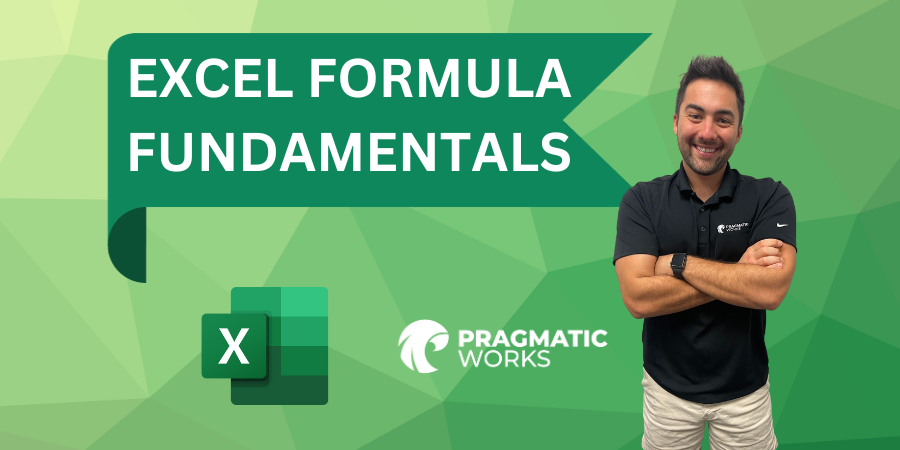Need help? Talk to an expert:(904) 638-5743

Private Training

Instructors

• Pivot Table Basics
• New Slicer Visual in Power BI
• Pivot Table Basics
• New Slicer Visual in Power BI

# Excel Formula Fundamentals#### Introduction

Get ready to embark on a journey into the world of Excel formulas with Greg Trzeciak, an expert trainer from Pragmatic Works. In this beginner-level series, Greg promises to demystify Excel and equip you with the skills to navigate and utilize its powerful formula features.

#### Understanding Averages

• Greg kicks off the series by explaining the concept of averages.
• Average, as he describes it, involves dividing the total of values by the number of those values.
• Demonstrates calculating the average using the formula bar by typing "average," selecting the data range, and closing with an enter.
• Greg showcases flexibility, mentioning various ways to achieve the same result based on user preference.

#### Exploring Medians

• Greg smoothly transitions to medians, highlighting its function in finding the middle number in an ordered list.
• Demonstrates calculating the median using the formula bar and emphasizes the importance of pressing "tab" for accuracy.
• Explains how the median changes in scenarios with an odd versus even number of data points.

#### Cracking the Mode

• Next up is the mode, the value that appears most frequently in a dataset.
• Greg uses the formula bar to initiate the mode calculation, showcasing the flexibility of Excel functions.
• Demonstrates the process of selecting the range and closing parentheses for a complete formula.
• A real-world example from "Greg's Rodeo Eats" reveals the best-selling menu items.

#### Summing It Up

• Greg introduces the sum function, emphasizing its simplicity in calculating the total of a set of numbers.
• Demonstrates two different methods for calculating the sum: using the formula bar and working directly in the cell.
• Introduces the convenient AutoSum feature, located in the Home tab under Editing, simplifying the sum calculation process.
Encourages users to explore different methods based on personal preferences.

#### Extra Credit: AutoSum Feature

• Greg goes above and beyond by introducing the AutoSum feature.
• Shows how AutoSum, located in the Editing section of the Home tab, can quickly calculate the sum of a selected range.
• Highlights the user-friendly nature of AutoSum for those seeking a more straightforward approach to sum calculations.

#### Conclusion

Greg's engaging tutorial serves as an excellent foundation for beginners venturing into Excel formulas. From averages to modes and AutoSum, he provides a comprehensive overview, ensuring that even first-time Excel users gain confidence in manipulating data. Stay tuned for more from Greg Trzeciak as he guides you through the exciting world of Excel in upcoming episodes and sign up for the Pragmatic Works' on-demand learning platform for access to even more exclusive classes.